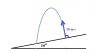# Simple question on 2d projectiles

• HMPARTICLE
In summary, the stone is shot at an angle of 10 degrees to the horizontal, and the time it takes to hit the ground is 0.32 seconds.f

## Homework Statement

A catapult projects a stone in the normal direction to a playground which slopes at a angle of 10 degrees to the horizontal.
The initial speed of the stone is 18 m/s. calculate the range parallel to the playground.

the usual ones!

## The Attempt at a Solution

My first equation is v = 18cos10i + 18sin10j
using v = u + at in the y direction with a = -9.8m/s^2 and v = 0 u =18sin10
using this i find that the time that the stone hits the ground is t = 0.32 seconds
now in the x direction x = vt since we ignore air resistance
i obtain 18cos10(0.32) = 5. 8 m

the answer in the back of the book is 11.8 m.

so i am wrong. i think i have interpreted the question incorrectly!
but i have tried setting the angle to 80 degrees, but that came out horribly wrong

My first equation is v = 18cos10i + 18sin10j
Where i is horizontal? No. A useful check is to consider what cos theta and sin theta give when theta = 0. In this case, that means a horizontal playground, so the stone goes straight up.
using v = u + at in the y direction with a = -9.8m/s^2 and v = 0 u =18sin10
When considering the final speed of a projectile, you must take the speed just before it hits whatever it hits. The speed after it has landed is irrelevant.

•HMPARTICLE
My first equation is v = 18cos10i + 18sin10j
Does the initial velocity vector make a 10 degree angle to the horizontal?
using v = u + at in the y direction with a = -9.8m/s^2 and v = 0 u =18sin10
using this i find that the time that the stone hits the ground is t = 0.32 seconds

Looks like you are finding the time at which the vertical component of velocity is zero. Is this the time that you want to use for when the stone returns to the ground?

Also, note that the stone does not return to the same vertical height as it started due to the tilt of the playground.

I think things will work out more easily if you choose your coordinate system such that the x-axis runs down along the slope of the playground and the y-axis is perpendicular to the ground of the playground.

#### Attachments

•Proj slope.png
1.2 KB · Views: 374
•HMPARTICLE
if i was to use the suggested co-ordinate system wouldn't the cannon just fire at a 90 degree angle?

if i was to use the suggested co-ordinate system wouldn't the cannon just fire at a 90 degree angle?

Yes, the cannon would fire at a 90 degree angle with respect to the x-axis running along the slope. If you do decide to choose your x-axis parallel to the slope and the y-axis perpendicular to the slope, then you will want to think about the x and y components of acceleration of the stone.

•HMPARTICLE
DONE! Thank you so much guys!